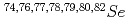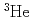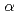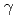Neutron nuclear data onhave been calculated for the evaluated nuclear data library JENDL-4. Simultaneously calculated are the total, elastic and inelastic scattering, (n,p), (n,d), (n,t), (n,), (n,), (n,np), (n,nd), (n,n), (n,2n), (n,3n) reaction cross sections, the angular distributions of emitted particles, and the energy distributions of emitted particles and-rays. The statistical model was applied to calculate these quantities. Coupled-channel optical model parameters were used for neutrons. Preequilibrium and direct-reaction processes were taken into account in addition to the compound process. The present calculations are consistent with available experimental data. The calculated results are compiled into JENDL-4.